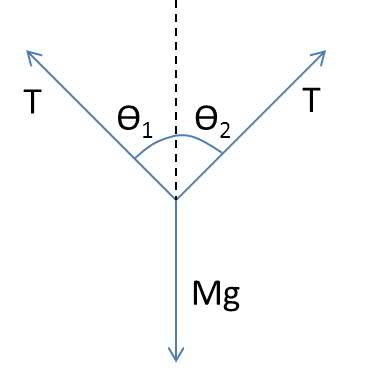# Forces-vector diagram triangles

when a cable car is stationary,it is in equilibrium.So the forces acting on it forms a closed triangle.(the vector diagram) Why is the triangle an isosceles triangle?

I did this question on my paper and stumbled across this on the answers,couldnt figure out why
hope someone could enlighten me!

## Answers and Replies

HallsofIvy
Science Advisor
Homework Helper
I'm not the best person to be answering physics questions but I don't see that it is. Am I misunderstanding the question? I have the weight of the car, straight downward, a force due to the cable, perpendicular to the cable, and a component of force parallel to the cable, holding the car stationary. I do not see that this is an isosceles triangle.

hm..in your scenario it doesnt seem to be stationery to me,seems like the resultant force isnt 0 and is towards the opposite direction of the parallel force to the cable you mentioned. I'm referring to 3 forces that act on the cable car(weight and 2 tension forces at certain degrees to vertical and horizontal) to form a closed triangle since the cable car is in equilibrium. I can't get why the answer says when it is in equilibrium the triangle is isosceles.

Make a Free-Body diagram, assume that the tension forces makes angles θ1 and θ2. Observe that the sin components equals (as the cable car is stationary) from where you can get θ12. You can then proceed further.θ1 is not necessarily equal to θ2 in the way the OP has presented the question. You can have tension at different angles and still keep it in equilibrium.

For example, call the two tensions T1=1N acting -30 degrees from the vertical and T2=(1/√3)N acting at 60 degrees from the vertical with the cable car having a weight (2/√3)N.

If there is the extra condition that both the tensions are equal, then yes, you could say they form an isoceles triangle.